### Home > A2C > Chapter 9 > Lesson 9.3.3 > Problem9-171

9-171.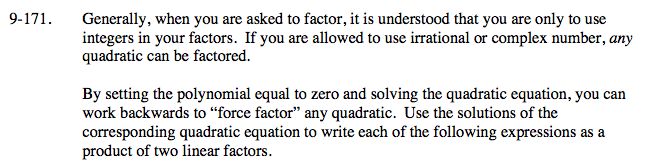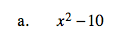Treat this as a difference of squares.

$(x+\sqrt{10})(x-\sqrt{10})$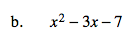$\left(x-\frac{3+\sqrt{37}}{2}\right)\left(x-\frac{3-\sqrt{37}}{2}\right)$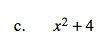Using complex numbers, this can be treated as a difference of squares.

x2 + 4 = x2 − (−4)

(x + 2i)(x − 2i)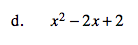See part (b).